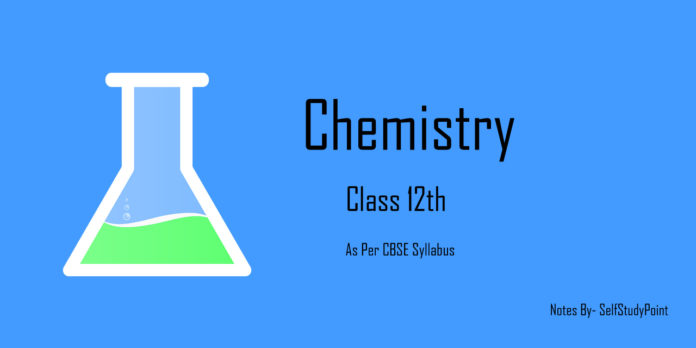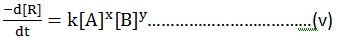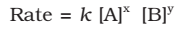# Rate expression and rate constant

Consider a general reaction

aA + bB → cC + dD

Where, a, b, c, and d are the stoichiometric coefficient of reactants and products.

The rate expression for this reaction is: –

The rate is directly proportional to [A]x [B]y ….(iii)

Where component x & y may or may not be equal to the stoichiometric coefficient (a & b) of the reactants

Also, Rate = k [A]x[B]y …..(iv)This form of equation (v) is known as differential rate equation, where k is proportionality constant called rate constant. And the equation (iii) which relates the rate of a reaction to the concentration of reactants is called Rate law or rate expression.

# Order of a Reaction

The sum of powers of the concentration of the reactants in the rate law expression is called the order of that chemical reaction.

Order of a reaction can be 0, 1, 2, 3 and even a fraction. A zero-order reaction means that the rate of reaction is independent of the concentration of reactants.## Units of the rate constant